Figures for "Duotone Truchet-like Tilings"

Cameron Browne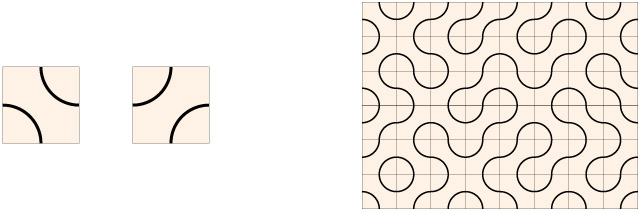Figure 1. Truchet-like tile and a Truchet-like tiling.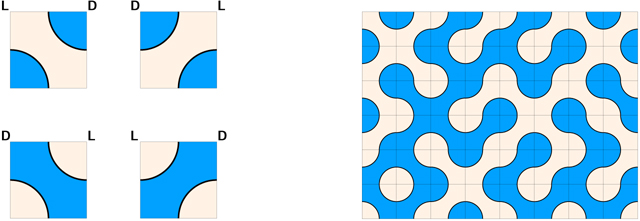Figure 2. Duotone Truchet-like tiles.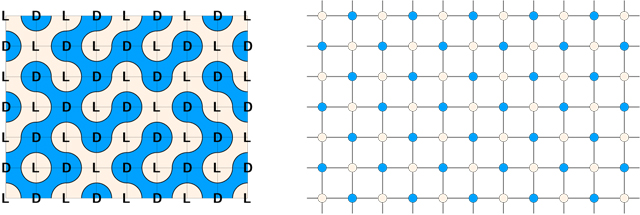Figure 3. Parity labels and parity graph.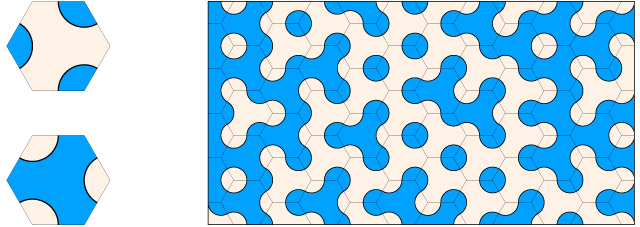Figure 4. Duotone Truchet-like hexagons.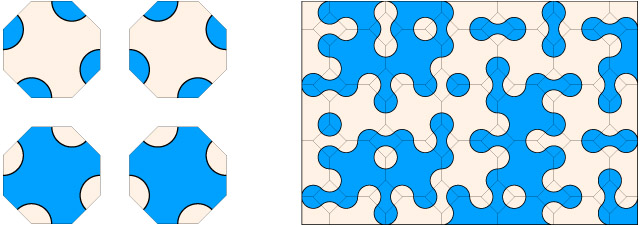Figure 5. Duotone Truchet-like octagons (4.4.8 tiling with squares).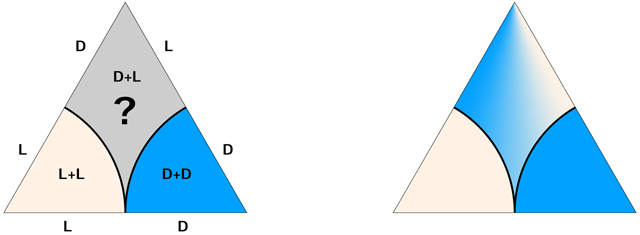Figure 6. Parity problem with duotone triangles.Figure 7. Duotone Truchet-like triangles.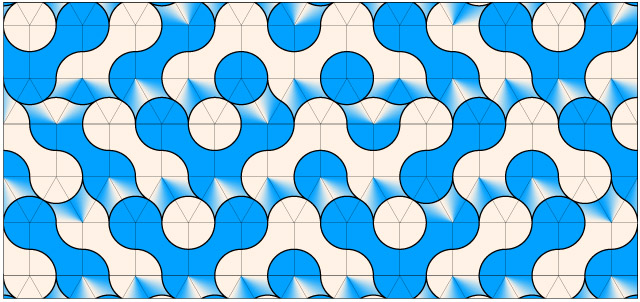Figure 8. Duotone squares and triangles (3.3.3.4.4 tiling).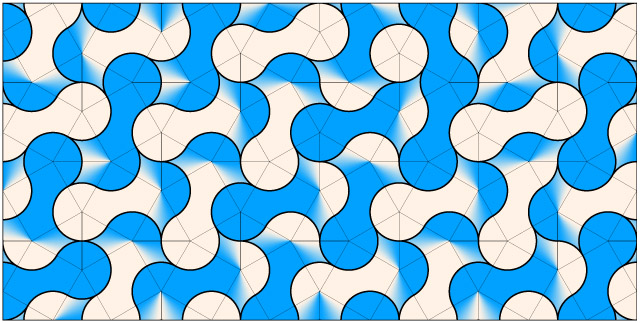Figure 9. Duotone squares and triangles (3.3.4.3.4 tiling).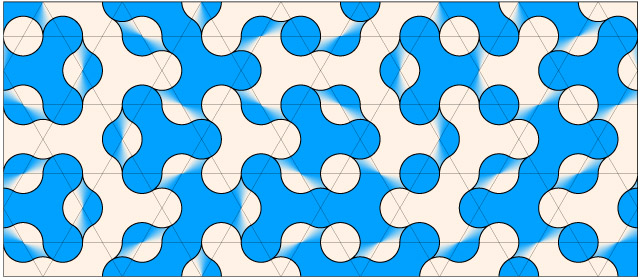Figure 10. Duotone hexagons and triangles (3.6.3.6 tiling).Figure 11. Duotone hexagons and triangles (3.3.3.3.6 tiling).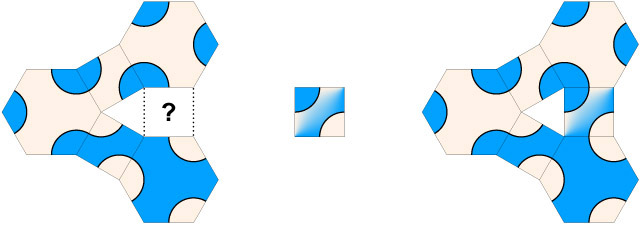Figure 12. Parity problem with the 3.4.6.4 tiling.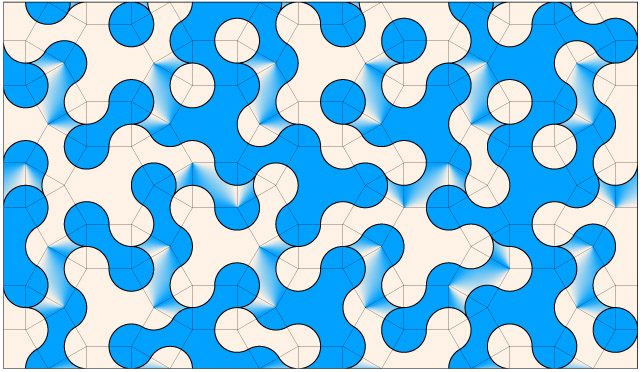Figure 13. Duotone hexagons, squares and triangles (3.4.6.4.tiling).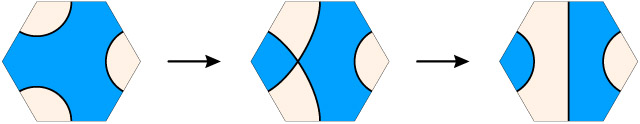Figure 14. Derivation of an arch tile.Figure 15. Three rotations of an arch tile and an arch tiling.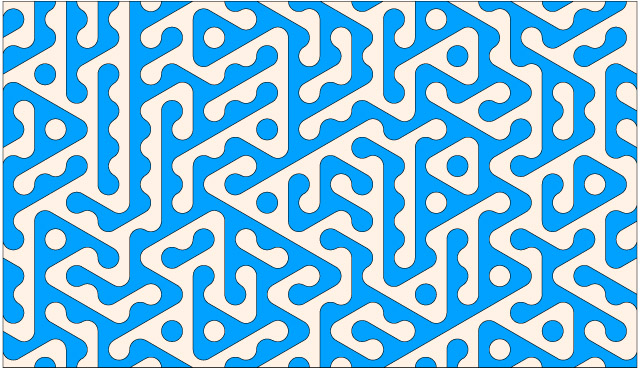Figure 16. Arch tiling with tile borders removed.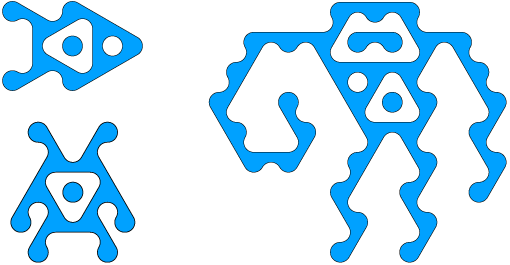Figure 17. Arch tile artwork: fish, monkey and Palagonian.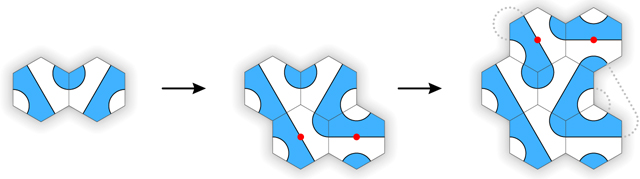Figure 18. A short game of Palago.